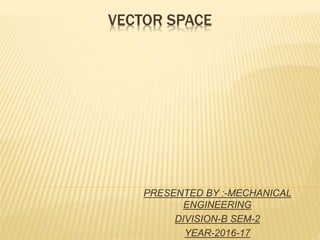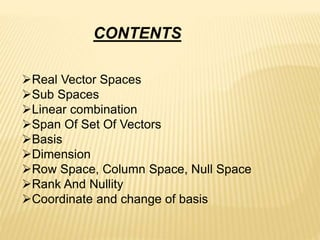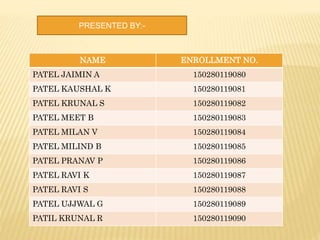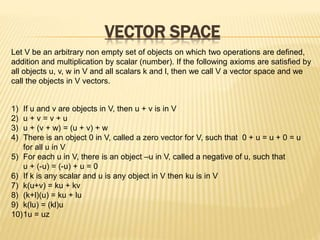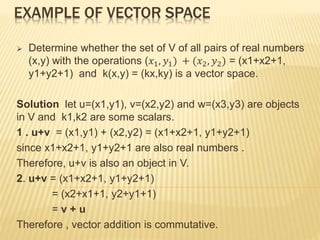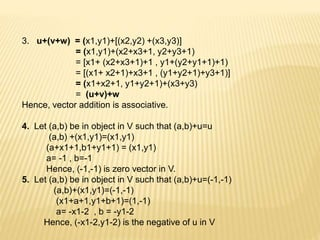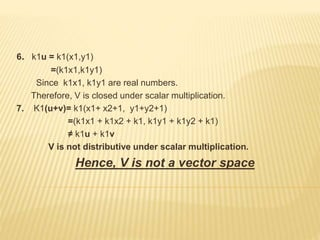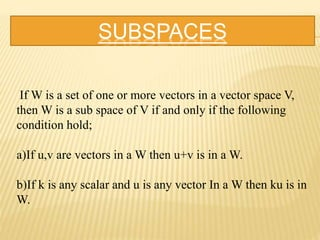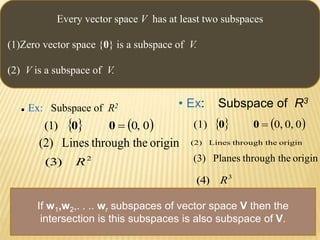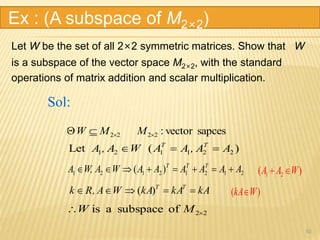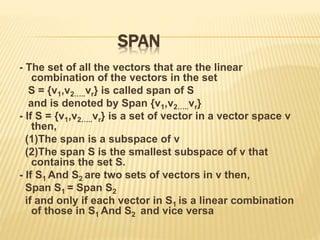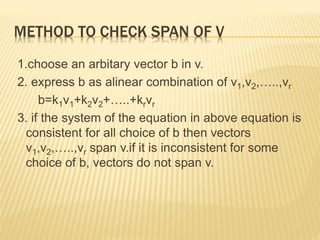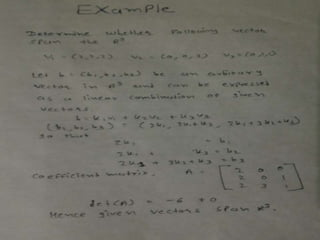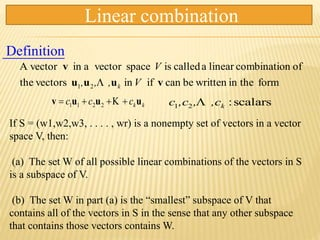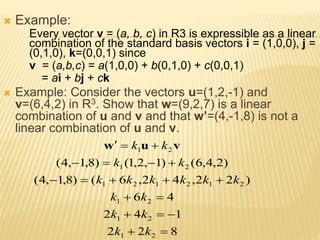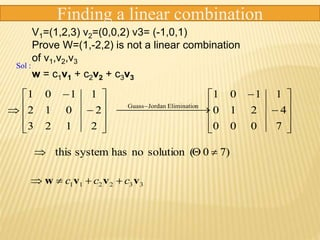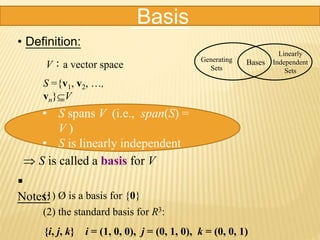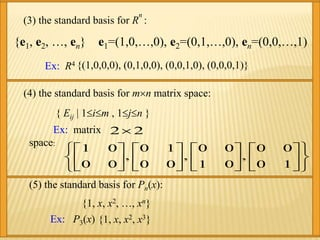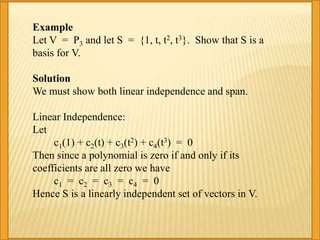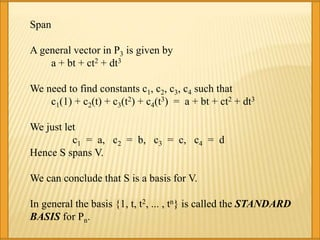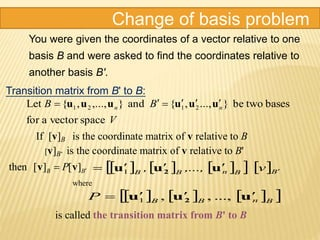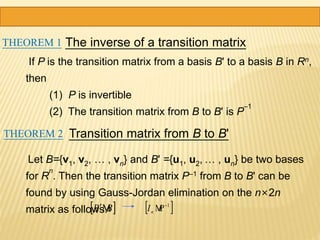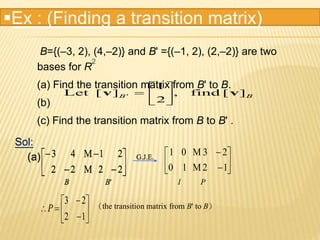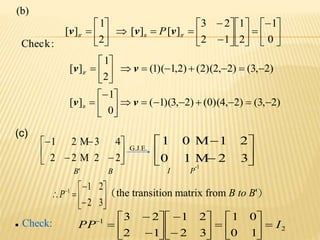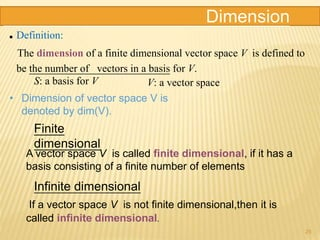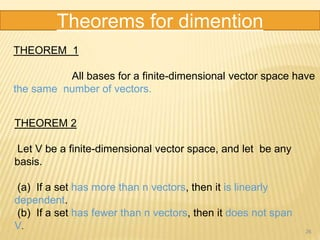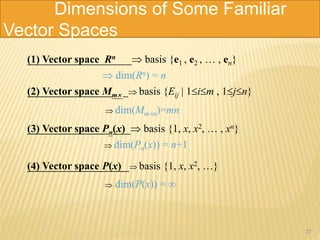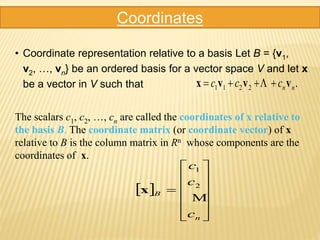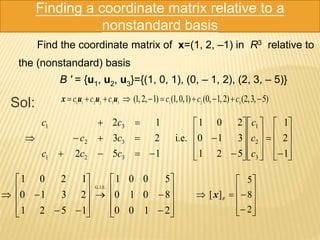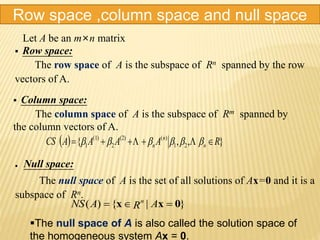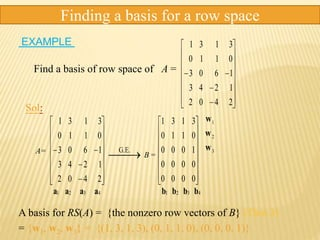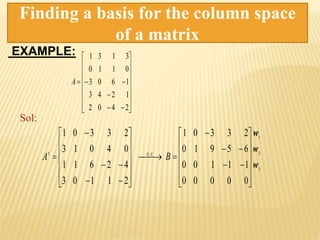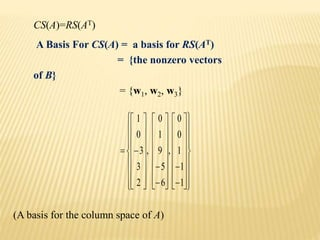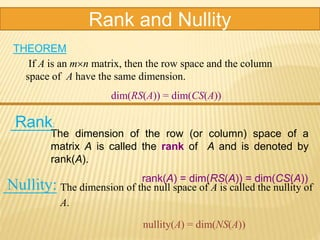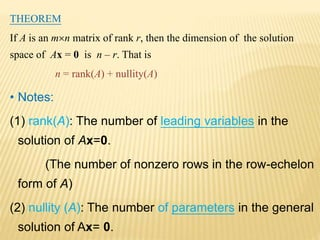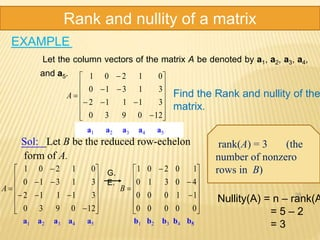1 de 37

### Vector space

1. VECTOR SPACE PRESENTED BY :-MECHANICAL ENGINEERING DIVISION-B SEM-2 YEAR-2016-17
2. Real Vector Spaces Sub Spaces Linear combination Span Of Set Of Vectors Basis Dimension Row Space, Column Space, Null Space Rank And Nullity Coordinate and change of basis CONTENTS
3. NAME ENROLLMENT NO. PATEL JAIMIN A 150280119080 PATEL KAUSHAL K 150280119081 PATEL KRUNAL S 150280119082 PATEL MEET B 150280119083 PATEL MILAN V 150280119084 PATEL MILIND B 150280119085 PATEL PRANAV P 150280119086 PATEL RAVI K 150280119087 PATEL RAVI S 150280119088 PATEL UJJWAL G 150280119089 PATIL KRUNAL R 150280119090 PRESENTED BY:-
4. VECTOR SPACE Let V be an arbitrary non empty set of objects on which two operations are defined, addition and multiplication by scalar (number). If the following axioms are satisfied by all objects u, v, w in V and all scalars k and l, then we call V a vector space and we call the objects in V vectors. 1) If u and v are objects in V, then u + v is in V 2) u + v = v + u 3) u + (v + w) = (u + v) + w 4) There is an object 0 in V, called a zero vector for V, such that 0 + u = u + 0 = u for all u in V 5) For each u in V, there is an object –u in V, called a negative of u, such that u + (-u) = (-u) + u = 0 6) If k is any scalar and u is any object in V then ku is in V 7) k(u+v) = ku + kv 8) (k+l)(u) = ku + lu 9) k(lu) = (kl)u 10)1u = uz
5. EXAMPLE OF VECTOR SPACE  Determine whether the set of V of all pairs of real numbers (x,y) with the operations (𝑥1, 𝑦1) + (𝑥2, 𝑦2) = (x1+x2+1, y1+y2+1) and k(x,y) = (kx,ky) is a vector space. Solution let u=(x1,y1), v=(x2,y2) and w=(x3,y3) are objects in V and k1,k2 are some scalars. 1 . u+v = (x1,y1) + (x2,y2) = (x1+x2+1, y1+y2+1) since x1+x2+1, y1+y2+1 are also real numbers . Therefore, u+v is also an object in V. 2. u+v = (x1+x2+1, y1+y2+1) = (x2+x1+1, y2+y1+1) = v + u Therefore , vector addition is commutative.
6. 3. u+(v+w) = (x1,y1)+[(x2,y2) +(x3,y3)] = (x1,y1)+(x2+x3+1, y2+y3+1) = [x1+ (x2+x3+1)+1 , y1+(y2+y1+1)+1) = [(x1+ x2+1)+x3+1 , (y1+y2+1)+y3+1)] = (x1+x2+1, y1+y2+1)+(x3+y3) = (u+v)+w Hence, vector addition is associative. 4. Let (a,b) be in object in V such that (a,b)+u=u (a,b) +(x1,y1)=(x1,y1) (a+x1+1,b1+y1+1) = (x1,y1) a= -1 , b=-1 Hence, (-1,-1) is zero vector in V. 5. Let (a,b) be in object in V such that (a,b)+u=(-1,-1) (a,b)+(x1,y1)=(-1,-1) (x1+a+1,y1+b+1)=(1,-1) a= -x1-2 , b = -y1-2 Hence, (-x1-2,y1-2) is the negative of u in V
7. 6. k1u = k1(x1,y1) =(k1x1,k1y1) Since k1x1, k1y1 are real numbers. Therefore, V is closed under scalar multiplication. 7. K1(u+v)= k1(x1+ x2+1, y1+y2+1) =(k1x1 + k1x2 + k1, k1y1 + k1y2 + k1) ≠ k1u + k1v V is not distributive under scalar multiplication. Hence, V is not a vector space
8. SUBSPACES If W is a set of one or more vectors in a vector space V, then W is a sub space of V if and only if the following condition hold; a)If u,v are vectors in a W then u+v is in a W. b)If k is any scalar and u is any vector In a W then ku is in W.
9. originhethrough tLines(2) 9 Every vector space V has at least two subspaces (1)Zero vector space {0} is a subspace of V. (2) V is a subspace of V.  Ex: Subspace of R2    00,(1) 00 originhethrough tLines(2) 2 (3) R • Ex: Subspace of R3 originhethrough tPlanes(3) 3 (4) R    00,0,(1) 00 If w1,w2,. . .. wr subspaces of vector space V then the intersection is this subspaces is also subspace of V.
10. Let W be the set of all 2×2 symmetric matrices. Show that W is a subspace of the vector space M2×2, with the standard operations of matrix addition and scalar multiplication. 10 sapcesvector:2222  MMW Sol: )(Let 221121 AA,AAWA,A TT  )( 21212121 AAAAAAWAW,A TTT  )( kAkAkAWA,Rk TT  22ofsubspaceais  MW )( 21 WAA  )( WkA Ex : (A subspace of M2×2)
11. SPAN - The set of all the vectors that are the linear combination of the vectors in the set S = {v1,v2…..vr} is called span of S and is denoted by Span {v1,v2…..vr} - If S = {v1,v2…..vr} is a set of vector in a vector space v then, (1)The span is a subspace of v (2)The span S is the smallest subspace of v that contains the set S. - If S1 And S2 are two sets of vectors in v then, Span S1 = Span S2 if and only if each vector in S1 is a linear combination of those in S1 And S2 and vice versa
12. METHOD TO CHECK SPAN OF V 1.choose an arbitary vector b in v. 2. express b as alinear combination of v1,v2,…..,vr b=k1v1+k2v2+…..+krvr 3. if the system of the equation in above equation is consistent for all choice of b then vectors v1,v2,…..,vr span v.if it is inconsistent for some choice of b, vectors do not span v.
13. kkccc uuuv  2211 formin thewrittenbecanifinvectorsthe ofncombinatiolinearacalledisspacevectorainA vector 21 vuuu v V,,, V k Definition scalars:21 k,c,,cc  Linear combination If S = (w1,w2,w3, . . . . , wr) is a nonempty set of vectors in a vector space V, then: (a) The set W of all possible linear combinations of the vectors in S is a subspace of V. (b) The set W in part (a) is the “smallest” subspace of V that contains all of the vectors in S in the sense that any other subspace that contains those vectors contains W.
14.  Example: Every vector v = (a, b, c) in R3 is expressible as a linear combination of the standard basis vectors i = (1,0,0), j = (0,1,0), k=(0,0,1) since v = (a,b,c) = a(1,0,0) + b(0,1,0) + c(0,0,1) = ai + bj + ck  Example: Consider the vectors u=(1,2,-1) and v=(6,4,2) in R3. Show that w=(9,2,7) is a linear combination of u and v and that w’=(4,-1,8) is not a linear combination of u and v. 822 142 46 )22,42,6()8,1,4( )2,4,6()1,2,1()8,1,4( 21 21 21 212121 21 21       kk kk kk kkkkkk kk kk vuw
15.              2123 2012 1101    nEliminatioJordanGuass             7000 4210 1101 )70(solutionnohassystemthis   332211 vvvw ccc  V1=(1,2,3) v2=(0,0,2) v3= (-1,0,1) Prove W=(1,-2,2) is not a linear combination of v1,v2,v3 w = c1v1 + c2v2 + c3v3 Finding a linear combination Sol :
16. Basis • Definition: V：a vector space Generating Sets Bases Linearly Independent Sets  S is called a basis for V S ={v1, v2, …, vn}V • S spans V (i.e., span(S) = V ) • S is linearly independent (1) Ø is a basis for {0} (2) the standard basis for R3: {i, j, k} i = (1, 0, 0), j = (0, 1, 0), k = (0, 0, 1)  Notes:
17. (3) the standard basis for R n : {e1, e2, …, en} e1=(1,0,…,0), e2=(0,1,…,0), en=(0,0,…,1) Ex: R4 {(1,0,0,0), (0,1,0,0), (0,0,1,0), (0,0,0,1)} Ex: matrix space:                               10 00 , 01 00 , 00 10 , 00 01 22 (4) the standard basis for mn matrix space: { Eij | 1im , 1jn } (5) the standard basis for Pn(x): {1, x, x2, …, xn} Ex: P3(x) {1, x, x2, x3}
18. Example Let V = P3 and let S = {1, t, t2, t3}. Show that S is a basis for V. Solution We must show both linear independence and span. Linear Independence: Let c1(1) + c2(t) + c3(t2) + c4(t3) = 0 Then since a polynomial is zero if and only if its coefficients are all zero we have c1 = c2 = c3 = c4 = 0 Hence S is a linearly independent set of vectors in V.
19. Span A general vector in P3 is given by a + bt + ct2 + dt3 We need to find constants c1, c2, c3, c4 such that c1(1) + c2(t) + c3(t2) + c4(t3) = a + bt + ct2 + dt3 We just let c1 = a, c2 = b, c3 = c, c4 = d Hence S spans V. We can conclude that S is a basis for V. In general the basis {1, t, t2, ... , tn} is called the STANDARD BASIS for Pn.
20. Change of basis problem You were given the coordinates of a vector relative to one basis B and were asked to find the coordinates relative to another basis B'. Transition matrix from B' to B: V BB nn spacevectorafor basestwobe}...,,{nda},...,,{etL 2121 uuuuuu  BB P  ][][hent vv         BBnBB v,...,,  1 uuu 2       BnBB P uuu 2  ...,,,1 where is called the transition matrix from B' to B If [v]B is the coordinate matrix of v relative to B [v]B‘ is the coordinate matrix of v relative to B'
21. If P is the transition matrix from a basis B' to a basis B in Rn, then (1) P is invertible (2) The transition matrix from B to B' is P –1 THEOREM 1 The inverse of a transition matrix THEOREM 2 Let B={v1, v2, … , vn} and B' ={u1, u2, … , un} be two bases for R n . Then the transition matrix P–1 from B to B' can be found by using Gauss-Jordan elimination on the n×2n matrix as follows. Transition matrix from B to B'  BB   1 PIn 
22. B={(–3, 2), (4,–2)} and B' ={(–1, 2), (2,–2)} are two bases for R 2 (a) Find the transition matrix from B' to B. (b) (c) Find the transition matrix from B to B' . BB ][find, 2 1 ][Let ' vv        Ex : (Finding a transition matrix) Sol:           22 21 22 43           12 23 10 01  G.J.E. B B' I P          12 23 P (the transition matrix from B' to B) (a) Sol:           22 21 22 43   G.J.E. B B' (a)
23.                             0 1 2 1 12 23 ][][ 2 1 ][ BBB P vvv (b)           22 43 22 21           32 21 10 01   G.J.E. B' B I P -1 (the transition matrix from B to B')          32 211 P  Check: 2 1 10 01 32 21 12 23 IPP                        )2,3()2,4)(0()2,3)(1( 0 1 ][ )2,3()2,2)(2()2,1)(1( 2 1 ][ :Check               vv vv B B (c)
24. 25 Dimension  Definition: The dimension of a finite dimensional vector space V is defined to be the number of vectors in a basis for V. V: a vector spaceS: a basis for V Finite dimensional A vector space V is called finite dimensional, if it has a basis consisting of a finite number of elements Infinite dimensional If a vector space V is not finite dimensional,then it is called infinite dimensional. • Dimension of vector space V is denoted by dim(V).
25. 26 Theorems for dimention THEOREM 1 All bases for a finite-dimensional vector space have the same number of vectors. THEOREM 2 Let V be a finite-dimensional vector space, and let be any basis. (a) If a set has more than n vectors, then it is linearly dependent. (b) If a set has fewer than n vectors, then it does not span V.
26. 27 Dimensions of Some Familiar Vector Spaces (1) Vector space Rn  basis {e1 , e2 ,  , en} (2) Vector space Mm  basis {Eij | 1im , 1jn} (3) Vector space Pn(x)  basis {1, x, x2,  , xn} (4) Vector space P(x)  basis {1, x, x2, }  dim(Rn) = n  dim(Mmn)=mn  dim(Pn(x)) = n+1  dim(P(x)) = 
27. Coordinates • Coordinate representation relative to a basis Let B = {v1, v2, …, vn} be an ordered basis for a vector space V and let x be a vector in V such that .2211 nnccc vvvx   The scalars c1, c2, …, cn are called the coordinates of x relative to the basis B. The coordinate matrix (or coordinate vector) of x relative to B is the column matrix in Rn whose components are the coordinates of x.                n B c c c  2 1 x
28. Find the coordinate matrix of x=(1, 2, –1) in R3 relative to the (nonstandard) basis B ' = {u1, u2, u3}={(1, 0, 1), (0, – 1, 2), (2, 3, – 5)} Sol: 2100 8010 5001 1521 2310 1201 E.G.J.                         )5,3,2()2,1,0()1,0,1()1,2,1( 321332211  cccccc uuux                                       1 2 1 521 310 201 i.e. 152 23 12 3 2 1 321 32 31 c c c ccc cc cc              2 8 5 ][ B x Finding a coordinate matrix relative to a nonstandard basis
29.  Row space: The row space of A is the subspace of Rn spanned by the row vectors of A.  Column space: The column space of A is the subspace of Rm spanned by the column vectors of A.   },,{ 21 )((2) 2 (1) 1 RAAAACS n n n    }|{)( 0xx  ARANS n  Null space: The null space of A is the set of all solutions of Ax=0 and it is a subspace of Rn. Let A be an m×n matrix Row space ,column space and null space The null space of A is also called the solution space of the homogeneous system Ax = 0.
30. Find a basis of row space of A =                    2402 1243 1603 0110 3131 Sol:                    2402 1243 1603 0110 3131 A=                 0000 0000 1000 0110 3131 3 2 1 w w w B = .E.G bbbbaaaa 43214321 Finding a basis for a row space EXAMPLE A basis for RS(A) = {the nonzero row vectors of B} (Thm 3) = {w1, w2, w3} = {(1, 3, 1, 3), (0, 1, 1, 0), (0, 0, 0, 1)}
31. Finding a basis for the column space of a matrix                    2402 1243 1603 0110 3131 A Sol: 3 2 1 .. 00000 11100 65910 23301 21103 42611 04013 23301 w w w                                 BA EGT EXAMPLE:
32. CS(A)=RS(AT) (A basis for the column space of A) A Basis For CS(A) = a basis for RS(AT) = {the nonzero vectors of B} = {w1, w2, w3}                                                                      1 1 1 0 0 , 6 5 9 1 0 , 2 3 3 0 1
33. Rank and Nullity If A is an mn matrix, then the row space and the column space of A have the same dimension. dim(RS(A)) = dim(CS(A)) THEOREM The dimension of the row (or column) space of a matrix A is called the rank of A and is denoted by rank(A). rank(A) = dim(RS(A)) = dim(CS(A)) Rank: Nullity: The dimension of the null space of A is called the nullity of A. nullity(A) = dim(NS(A))
34. THEOREM If A is an mn matrix of rank r, then the dimension of the solution space of Ax = 0 is n – r. That is n = rank(A) + nullity(A) • Notes: (1) rank(A): The number of leading variables in the solution of Ax=0. (The number of nonzero rows in the row-echelon form of A) (2) nullity (A): The number of parameters in the general solution of Ax= 0.
35. 36 Rank and nullity of a matrix Let the column vectors of the matrix A be denoted by a1, a2, a3, a4, and a5.                  120930 31112 31310 01201 A a1 a2 a3 a4 a5 EXAMPLE Find the Rank and nullity of the matrix. Sol: Let B be the reduced row-echelon form of A.                                  00000 11000 40310 10201 120930 31112 31310 01201 BA a1 a2 a3 a4 a5 b1 b2 b3 b4 b5 G. E. rank(A) = 3 (the number of nonzero rows in B) Nullity(A) = n – rank(A = 5 – 2 = 3
36. THANK YOU GUIDED BY:- MR. JAYDEV PATEL